## NCERT Solutions for Class 6 Maths Exercise 5.9

NCERT Solutions for Class 6 Maths Exercise 5.9 book solutions are available in PDF format for free download. These ncert book chapter wise questions and answers are very helpful for CBSE exam. CBSE recommends NCERT books and most of the questions in CBSE exam are asked from NCERT text books. Class 6 Maths chapter wise … Read more

## NCERT Solutions for Class 6 Maths Exercise 9.1

NCERT Solutions for Class 6 Maths Exercise 9.1 book solutions are available in PDF format for free download. These ncert book chapter wise questions and answers are very helpful for CBSE exam. CBSE recommends NCERT books and most of the questions in CBSE exam are asked from NCERT text books. Class 6 Maths chapter wise … Read more

## NCERT Solutions for Class 6 Maths Exercise 13.3

NCERT Solutions for Class 6 Maths Exercise 13.3 book solutions are available in PDF format for free download. These ncert book chapter wise questions and answers are very helpful for CBSE exam. CBSE recommends NCERT books and most of the questions in CBSE exam are asked from NCERT text books. Class 6 Maths chapter wise … Read more

## NCERT Solutions for Class 6 Maths Exercise 2.2

NCERT Solutions for Class 6 Maths Exercise 2.2 book solutions are available in PDF format for free download. These ncert book chapter wise questions and answers are very helpful for CBSE exam. CBSE recommends NCERT books and most of the questions in CBSE exam are asked from NCERT text books. Class 6 Maths chapter wise … Read more

## NCERT Solutions for Class 6 Maths Exercise 6.1

NCERT Solutions for Class 6 Maths Exercise 6.1 book solutions are available in PDF format for free download. These ncert book chapter wise questions and answers are very helpful for CBSE exam. CBSE recommends NCERT books and most of the questions in CBSE exam are asked from NCERT text books. Class 6 Maths chapter wise … Read more

## NCERT Solutions for Class 6 Maths Exercise 9.2

NCERT Solutions for Class 6 Maths Exercise 9.2 book solutions are available in PDF format for free download. These ncert book chapter wise questions and answers are very helpful for CBSE exam. CBSE recommends NCERT books and most of the questions in CBSE exam are asked from NCERT text books. Class 6 Maths chapter wise … Read more

## NCERT Solutions for Class 6 Maths Exercise 14.1

NCERT Solutions for Class 6 Maths Exercise 14.1 book solutions are available in PDF format for free download. These ncert book chapter wise questions and answers are very helpful for CBSE exam. CBSE recommends NCERT books and most of the questions in CBSE exam are asked from NCERT text books. Class 6 Maths chapter wise … Read more

## NCERT Solutions for Class 6 Maths Exercise 2.3

NCERT Solutions for Class 6 Maths Exercise 2.3 book solutions are available in PDF format for free download. These ncert book chapter wise questions and answers are very helpful for CBSE exam. CBSE recommends NCERT books and most of the questions in CBSE exam are asked from NCERT text books. Class 6 Maths chapter wise … Read more

## NCERT Solutions for Class 6 Maths Exercise 6.2

NCERT Solutions for Class 6 Maths Exercise 6.2 book solutions are available in PDF format for free download. These ncert book chapter wise questions and answers are very helpful for CBSE exam. CBSE recommends NCERT books and most of the questions in CBSE exam are asked from NCERT text books. Class 6 Maths chapter wise … Read more

## NCERT Solutions for Class 6 Maths Exercise 9.3

NCERT Solutions for Class 6 Maths Exercise 9.3 book solutions are available in PDF format for free download. These ncert book chapter wise questions and answers are very helpful for CBSE exam. CBSE recommends NCERT books and most of the questions in CBSE exam are asked from NCERT text books. Class 6 Maths chapter wise … Read more

## NCERT Solutions for Class 6 Maths Exercise 14.2

NCERT Solutions for Class 6 Maths Exercise 14.2 book solutions are available in PDF format for free download. These ncert book chapter wise questions and answers are very helpful for CBSE exam. CBSE recommends NCERT books and most of the questions in CBSE exam are asked from NCERT text books. Class 6 Maths chapter wise … Read more

## NCERT Solutions for Class 6 Maths Exercise 4.1

NCERT solutions for Maths Basic geometrical ideas## NCERT Solutions for Class 6 Maths Basic geometrical ideas

###### Question 1.Use the figure to name: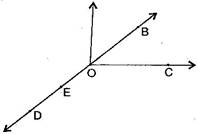(a)Five points

(b)A line

(c)Four rays

(d)Five line segments

(a) Five points are: O, B, C, D, E

(b) A line: {tex}\overline {{\text{DE}}} ,{/tex}{tex}\overline {{\text{DB}}} ,{/tex}{tex}\overline {{\text{OE}}} ,{/tex}{tex}\overline {{\text{OB}}} {/tex}

(c) Four rays: {tex}\overrightarrow {{\text{OD}}} ,{/tex}{tex}\overrightarrow {{\text{OE}}} ,{/tex}{tex}\overrightarrow {{\text{OC}}} ,{/tex}{tex}\overrightarrow {{\text{OB}}} {/tex}

(d) Four line segments: {tex}\overline {{\text{DE}}} ,{/tex}{tex}\overline {{\text{OE}}} ,{/tex}{tex}\overline {{\text{OC}}} ,{/tex}{tex}\overline {{\text{OB}}} ,{/tex}, {tex}\overline {{\text{OD}}} {/tex}

NCERT Solutions for Class 6 Maths Exercise 4.1

###### Question 2.Name the line given in all possible (twelve) ways, choosing only two letters at a time from the four given.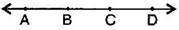Answer: {tex}\overline {{\text{AB}}} ,{/tex}{tex}\overline {{\text{AC}}} ,{/tex}{tex}\overline {{\text{AD}}} ,{/tex}{tex}\overline {{\text{BC}}} ,{/tex}{tex}\overline {{\text{BD}}} ,{/tex}{tex}\overline {{\text{CD}}} ,{/tex}{tex}\overline {{\text{BA}}} ,{/tex}{tex}\overline {{\text{CA}}} ,{/tex}{tex}\overline {{\text{DA}}} ,{/tex}{tex}\overline {{\text{CB}}} ,{/tex}{tex}\overline {{\text{DB}}} ,{/tex}{tex}\overline {{\text{DC}}} {/tex}

###### Question 3.Use the figure to name: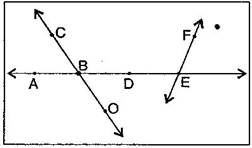(a)Line containing point E.

(b)Line passing through A.

(c)Line on which O lies.

(d)Two pairs of intersecting lines.

Answer: (a) A line containing E = {tex}\overline {{\text{AE}}} {/tex} or {tex}\overline {{\text{FE}}} {/tex}

(b) A line passing through A = {tex}\overline {{\text{AE}}} {/tex} or {tex}\overline {{\text{DE}}} {/tex}

(c) A line on which O lies = {tex}\overline {{\text{CO}}} {/tex} or {tex}\overline {{\text{OC}}} {/tex}

(d) Two pairs of intersecting lines are : {tex}\overline {{\text{AD}}} ,{/tex}{tex}\overline {{\text{CO}}} {/tex} and {tex}\overline {{\text{AE}}} {/tex}, {tex}\overline {{\text{FE}}} {/tex}

NCERT Solutions for Class 6 Maths Exercise 4.1

###### Question 4.How many lines can pass though:

(a) one given point?

(b) two given points

Answer: (a) Infinite number of lines can pass through one given point.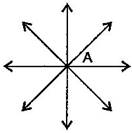(b) Only one line can pass through two given points.

###### Question 5.Draw a rough figure and label suitably in each of the following cases:

(a)Point P lies on {tex}\overline {{\text{AB}}} .{/tex}

(b) {tex}\overline {{\text{XY}}} {/tex} and {tex}\overline {{\text{PQ}}} {/tex} intersect at M.

(c) Line {tex}l{/tex} contains E and F but not D.

(d) {tex}\overline {{\text{OP}}} {/tex} and {tex}\overline {{\text{OQ}}} {/tex} meet at O.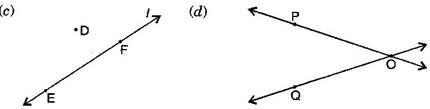NCERT Solutions for Class 6 Maths Exercise 4.1

###### Question 6.Consider the following figure of line {tex}\overline {{\text{MN}}} {/tex}. Say whether following statements are true or false in the context of the given figure:

Read moreNCERT Solutions for Class 6 Maths Exercise 4.1

## NCERT Solutions for Class 6 Maths Exercise 6.3

NCERT Solutions for Class 6 Maths Exercise 6.3 book solutions are available in PDF format for free download. These ncert book chapter wise questions and answers are very helpful for CBSE exam. CBSE recommends NCERT books and most of the questions in CBSE exam are asked from NCERT text books. Class 6 Maths chapter wise … Read more

## NCERT Solutions for Class 6 Maths Exercise 9.4

NCERT Solutions for Class 6 Maths Exercise 9.4 book solutions are available in PDF format for free download. These ncert book chapter wise questions and answers are very helpful for CBSE exam. CBSE recommends NCERT books and most of the questions in CBSE exam are asked from NCERT text books. Class 6 Maths chapter wise … Read more

## NCERT Solutions for Class 6 Maths Exercise 14.3

NCERT Solutions for Class 6 Maths Exercise 14.3 book solutions are available in PDF format for free download. These ncert book chapter wise questions and answers are very helpful for CBSE exam. CBSE recommends NCERT books and most of the questions in CBSE exam are asked from NCERT text books. Class 6 Maths chapter wise … Read more

## NCERT Solutions for Class 6 Maths Exercise 4.2

NCERT Solutions for Class 6 Maths Exercise 4.2 book solutions are available in PDF format for free download. These ncert book chapter wise questions and answers are very helpful for CBSE exam. CBSE recommends NCERT books and most of the questions in CBSE exam are asked from NCERT text books. Class 6 Maths chapter wise … Read more

## NCERT Solutions for Class 6 Maths Exercise 7.1

NCERT Solutions for Class 6 Maths Exercise 7.1 book solutions are available in PDF format for free download. These ncert book chapter wise questions and answers are very helpful for CBSE exam. CBSE recommends NCERT books and most of the questions in CBSE exam are asked from NCERT text books. Class 6 Maths chapter wise … Read more

## NCERT Solutions for Class 6 Maths Exercise 10.1

NCERT Solutions for Class 6 Maths Exercise 10.1 book solutions are available in PDF format for free download. These ncert book chapter wise questions and answers are very helpful for CBSE exam. CBSE recommends NCERT books and most of the questions in CBSE exam are asked from NCERT text books. Class 6 Maths chapter wise … Read more

## NCERT Solutions for Class 6 Maths Exercise 14.4

NCERT Solutions for Class 6 Maths Exercise 14.4 book solutions are available in PDF format for free download. These ncert book chapter wise questions and answers are very helpful for CBSE exam. CBSE recommends NCERT books and most of the questions in CBSE exam are asked from NCERT text books. Class 6 Maths chapter wise … Read more

## NCERT Solutions for Class 6 Maths Exercise 4.3

NCERT Solutions for Class 6 Maths Exercise 4.3 book solutions are available in PDF format for free download. These ncert book chapter wise questions and answers are very helpful for CBSE exam. CBSE recommends NCERT books and most of the questions in CBSE exam are asked from NCERT text books. Class 6 Maths chapter wise … Read more